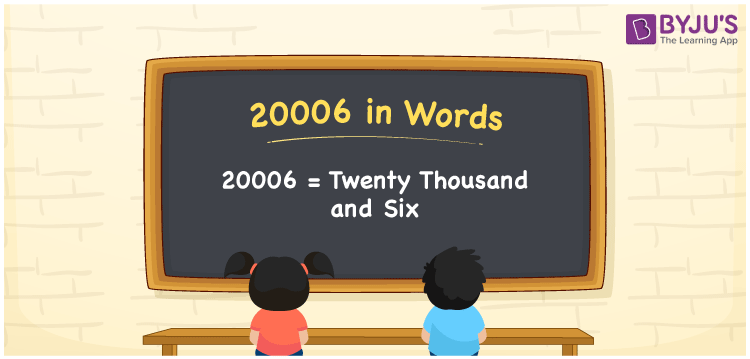# 20006 in Words

The number name of 20006 is twenty thousand and six. If Arun has saved Rs. 20006 in 7 months, it can be written as “Arun has saved Rs. Twenty thousand and six in seven months”. The number 20006 is a natural number and it has 5 digits. In this article, we are going to learn the technique of writing the number 20006 in words using the place value system in detail.

 20006 in Words: Twenty Thousand and Six. Twenty Thousand and Six in Numerical Form: 20006.

## 20006 in English Words## How to Write 20006 in Words?

Below is the place value table for the numeral 20006.

 Ten-thousands Thousands Hundreds Tens Ones 2 0 0 0 6

The expanded form of 20006 is as follows:

= 2 × Ten thousands + 0 × Thousand + 0 × Hundred + 0 × Ten + 6 × One

= 2 × 10000 + 0 × 1000 + 0 × 100 + 0 × 10 + 6 × 1

= 20000 + 6

= 20006

= Twenty thousand and six

Hence, 20006 in words is twenty thousand and six.

20006 in words – Twenty thousand and six

Is 20006 an odd number? – No

Is 20006 an even number? – Yes

Is 20006 a perfect square number? – No

Is 20006 a perfect cube number? – No

Is 20006 a prime number? – No

Is 20006 a composite number? – Yes

## Frequently Asked Questions on 20006 in Words

Q1

### How to spell 20006?

20006 in words is twenty thousand and six.

Q2

### Simplify 20000 + 6, and express it in words.

Simplifying 20000 + 6, we get 20006. Hence, 20006 in words is twenty thousand and six.

Q3

### How to write twenty thousand and six in numbers?

Twenty thousand and six in numbers is 20006.